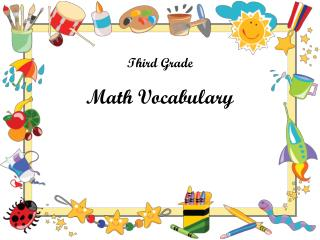DownloadDownload PresentationDownload Presentation- - - - - - - - - - - - - - - - - - - - - - - - - - - E N D - - - - - - - - - - - - - - - - - - - - - - - - - - -
##### Presentation Transcript

3. Properties of addition: *Identity Property-states that when you add zero to a number, the result is that number. Example 7 + 0 = 7 *Commutative property of addition- states that you can add two numbers in any order and get the same sum. Example 6 + 4 = 10 and 4 + 6 = 10 *Associative property- states that you can group addends in different ways & still get the same sum. Example (2+3)+1 = 2 + (3+1)

4. Place value – the value of each digit in a number, based on the location of the digit. decimal – a number with one or more digits to the right of of the decimal point.

5. Array – an arrangement of objects in rows and columns. column row Factor – a number that is multiplied by another number to find the product. This array represents 2 X 4 = 8 Product – the answer for a multiplication problem.

6. Properties of Multiplication • Associative property – states that when the grouping of factors is changed, the product remains the same. • Example (3 X 4) X 2 = 3 X (4 X 2) • Commutative property – states that you can two factors in any order and get the same product. • Example 4 X 7 = 28 and 7 X 4 = 28 • Identity property – states that the product of any number and one is that number. • Example 5 X 1 = 5 • Distributive property – states that multiplying a sum by a number is the same as multiplying each addend by the number, and then adding the products. • Example 3 X (4 + 2) = (3 X 4 ) + (3 X 2) • 3 X 6 = 12 + 6 • 18 = 18

7. Inverse Operations – opposite operations, or operations that undo each other, such as addition and subtraction or multiplication and division. *subtraction checks addition. * multiplication checks division 434 557 14 ÷ 7 = 2 because 7 X 2 = 14 +123 -123 557 434

8. What is division? Divide – to separate into equal groups; the opposite operation of multiplication. remainder Dividend – the number that is to be divided Divisor- the number that divides the dividend. Remainder – the amount left over when a number cannot be divided evenly.

9. Fraction- a number that names parts of a whole or part of a group. 1/3 Numerator – the part of a fraction above the line, which tells how many parts are being counted. Denominator – the part of a fraction below the line, which tells how many equal parts there are in the whole or in the group.

10. Triangles Scalene – a triangle in which no sides are equal. Isosceles – a triangle in which two sides are equal. Equilateral – a triangle that has three equal sides.

11. Area – the number of square units needed to cover a flat surface. Example : You can find area by counting the boxes. Area = 12 Or you can find area by using a formula. Area = l x w Area = 4 x 3 Area = 12

12. Perimeter – is (“The fence around the playground!”) the distance around a figure. You can find perimeter by counting the outside edges. Or you can find perimeter by using a formula. P = s + s + s + s P = 8 + 4 + 8 + 4 P = 24